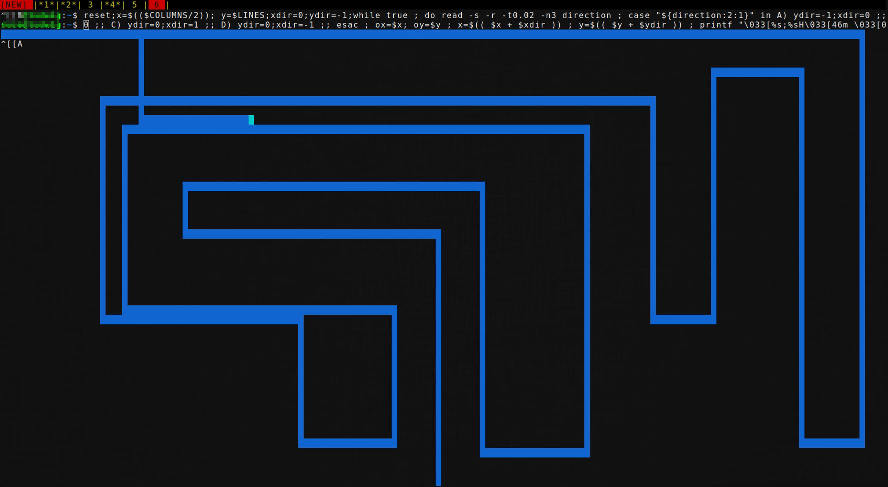# Tron Lightcycles or Etch-a-sketch gameCopy and paste the commands below into your bash shell. Or better yet read, type and learn. Upon running this will start moving your cursor from the bottom up and making a blue trail as you go. Use the arrow keys to move around. The first command doesn't have boundary or wall detection yet so keep your cursor in bounds.

reset;x=\$((\$COLUMNS/2)); y=\$LINES;xdir=0;ydir=-1;while true ; do read -s -r -t0.02 -n3 direction ; case "\${direction:2:1}" in A) ydir=-1;xdir=0 ;; B)ydir=1;xdir=0 ;; C) ydir=0;xdir=1 ;; D) ydir=0;xdir=-1 ;; esac ; ox=\$x; oy=\$y ; x=\$(( \$x + \$xdir )) ; y=\$(( \$y + \$ydir )) ; printf "\033[%s;%sH\033[46m \033[0m\033[%s;%sH\033[44m \033[0m\033[0;0H" \$y \$x \$oy \$ox ; sleep 0.01 ; done

Here is another version with wall collision detection. Using a bash array to keep track of where you have "drawn" a wall.

clear;x=\$((\$COLUMNS/2)); y=\$LINES;unset spacesused[*];declare -a spacesused;xdir=0;ydir=-1;while true ; do read -s -r -t0.02 -n3 direction ; case "\${direction:2:1}" in A) ydir=-1;xdir=0 ;; B)ydir=1;xdir=0 ;; C) ydir=0;xdir=1 ;; D) ydir=0;xdir=-1 ;; esac ; space=\$(( \$COLUMNS * \$y + \$x )) ; if [[ "\${spacesused[\$space]}" == "1" || \$x -gt \$COLUMNS || \$x -lt 0 || \$y -gt \$LINES || \$y -lt 0 ]]; then printf '\033[%d;%dHBOOM!\nEND OF LINE.\n' \$y \$x; break ; else spacesused[\$space]=1 ; fi ; ox=\$x; oy=\$y ; x=\$(( \$x + \$xdir )) ; y=\$(( \$y + \$ydir )) ; printf "\033[%s;%sH\033[46m \033[0m\033[%s;%sH\033[44m \033[0m\033[0;0H" \$y \$x \$oy \$ox ; sleep 0.01 ; done

And another version without the movement that is more like a paint program. Press an arrow key to begin. Press they 'p' key to turn off the brush (off by default). However turning it off will erase blocks you've drawn as you move over them. Press numbers 0 through 7 in order to set the color. 9 means the default background which will do the same thing as turning off the brush. 0 through 7 are the standard ANSI background colors.

reset;x=\$((\$COLUMNS/2));y=\$((\$LINES/2));xdir=0;ydir=-1;on=0;color=44;while true ; do read -srn1 direction ; case "\$direction" in A) ydir=-1;xdir=0 ;; B)ydir=1;xdir=0 ;; C) ydir=0;xdir=1 ;; D) ydir=0;xdir=-1 ;; p) on=\$(( \$on ^ 1 )) ; continue ;; [0-9]) color=4\$direction ; continue ;; *) continue ;; esac ; ox=\$x; oy=\$y ; x=\$(( \$x + \$xdir )) ; y=\$(( \$y + \$ydir )) ; if [[ "\$on" -eq "1" ]]; then paintcolor=\$color ; else paintcolor=49 ; fi ; printf "\033[%s;%sH\033[47m \033[0m\033[%s;%sH\033[%sm \033[0m\033[0;0H" \$y \$x \$oy \$ox \$paintcolor ; sleep 0.01 ; done